## General Question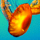# Factor completely:x^4+4

Asked by shared3 (921) March 16th, 2008

I got two answers, (x^2+2x+2)(x^2–2x+2) and (x^2+2i)(x^2–2i). I think both work. Are they both correct, and how do you get the second one?

Observing members: 0Composing members: 0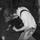yeah, they both work, I checked it. Unless I made some stupid mistake… I’m not sure how you get the imaginary one though I think it has something to do with factoring your first answer further and distributing it some funky way…. I’m trying to work it out now. :)

8lightminutesaway (1413)“Great Answer” (1) Flag as…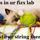Yes, the four solutions to x^4+4=0 are x=±1±i. So to factor this completely, you have:

x^4+4=(x+1+i)(x+1-i)(x-1+i)(x-1-i)

If you multiply the first two factors and the second two factors, you obtain

x^4+4=(x^2+2x+2)(x^2–2x+2)

Alternatively, if you multiply the first and last factor, and also the second and third, you get:

x^4+4=(x^2–2i)(x^2+2i)

You can, of course, write the factorization in a third way, that being

x^4+4=(x^2+2ix-2)(x^2–2ix-2).

chris (409)“Great Answer” (1) Flag as…Thanks for the responses!

How do you factor it completely? [How do you get (x+1+i)(x+1-i)(x-1+i)(x-1-i)]

shared3 (921)“Great Answer” (0) Flag as…you get that by factoring (x^2+2x+2) and (x^2–2x+2) using the quadratic formula for each.
For the first one:
(- 2 +- (2^2 – 4(1)(2))^1/2)/2
= (- 2 +-(4–8)^1/2))/2
= (- 2 +- (-4)^1/2)/2
=(- 2 +-2i)/2
=-1 +-i
and follow the same procedure for the other one. :)
@chris, how do u do the +- thing? lol

8lightminutesaway (1413)“Great Answer” (0) Flag as…@ 8lightminutesaway

If you have a mac, you get ± by pressing the (+/=) key while holding (option + shift). I’m afraid I can’t help for a pc.

chris (409)“Great Answer” (0) Flag as…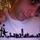A quicker way to do this equation in particular is to notice that you’re looking for the fourth roots of -4. When you find one, the others have to be that times the four fourth roots of one (namely, 1, -1, i, and -i). So once you find one, you’ve found them all.

There are quick ways to geometrically see where the fourth roots of -4 are if you look at the complex plane and use polar coordinates, but it’s a little tricky to describe without being able to draw pictures.

finkelitis (1907)“Great Answer” (0) Flag as…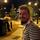Those are both correct answers. Good job!

JakePark (6)“Great Answer” (0) Flag as…or